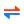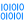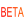Calculators list - IT/COMPUTERS
In this category you'll find calculators, tables or converters related to computers or generally speaking information technology (IT). Here you'll convert megabytes to kilobytes, see MTU paremeter for different kind of networks or divide your IP based LAN into subnets easily.
• ASCII-2-number converterThe converter of any ASCII or Unicode text to numbers. Allows for many format-related modifications of output. Numbers can be hexadecimal, decimal or binary. Setting separators (commas, linebreaks), dividing numbers to groups (ex. put "enter" after each 4 items) - is also "one-click-easy". Supports windows/linux/mac line-break codes.

• ASCII/HEX/HTML tableThe whole set of 127 ASCII characters. Table shows decimal (DEC), hexadecimal (HEX), octal (OCT) and binary (BIN) indexes, but also HTML entities (in 3 different formats), ANSI-C entities and ASCII descriptors.

• BASE64 encodingCalculator encodes given plain text using BASE64 algorithm or vice versa. Unicode characters are encoded as UTF-8 before BASE64 encoding is applied.

• BandwidthBandwidth units converter. Converts KB/s, Mbps etc. All known units of bandwidth in two different bases: 1000 and 1024.

• Binary prefixesBinary prefixes - kibi, mebi, gibi, tebi etc.

• Country ISO codesTable shows list of ISO 3166-1 and ISO 3166-2 codes assigned to different countries.

• Data storageData units converter. Converts bits, bytes, megabytes, gigabytes itd. All known units of data in two different bases: 1000 and 1024. This calculator handles some less known data units: nibbles, octets, pixels (24/48-bit), words, quads, long-words etc.

• Floating point numbersCalculator shows internal representation of IEEE 754 floating point numbers, as it is stored in computer's memory. You can see binary, hexadecimal and decimal dump of the number including split into sign, exponent and mantisa parts. Both single and double floating numbers are supported.

• HEX/DEC tableLookup table (4096 entries) for fast manual conversion of decimal to hex, or hex to decimal numbers.

• IP calculator (networks/subnets)• ISO 8859-2 (Latin-2) tableThe whole set of ISO 8859-2 (Latin-2) characters. Table shows decimal (DEC), hexadecimal (HEX), octal (OCT) and binary (BIN) indexes, but also HTML entities (in 3 different formats), ANSI-C entities, unicode (HEX, OCT and DEC) and textual descriptors.

• MTU tableMTU (Maximum Transmission Unit) values assigned to given network type.

• Number to wordsThis calculator converts given numbers to its textual forms e.g. 3.14 into three and fourteen hundredths.

• Polish PESEL generatorCalculator generates random PESEL numbers (unique ID assigned to each person in Poland) matching given criteria such as date of birth, age range or gender.

• Punycode encoding (IDN)Calculator encodes given domain using punycode algorithm or vice versa. Encoded domain name is ready to use with International Domain Name (IDN).

• Random numbersGenerates random numbers and texts. You can use this calculator to generate random passwords.

• Time: Date and time formatsCalculator converts date and time from one format to another. Supports number of calendars (Hebrew, Julian, Islamic, Persian, Indian national, Kurdish, Afgan, Mayan) and also computer time (UNIX time and Excel time).

• Top-level domainsTable shows common list of top-level internet domains such as .com, .net or related to given region such as .pl or .de.

• Words counterCalculator finds out the number of words, characters, sentences, paragraphs, keywords density etc. in a given text.

JavaScript failed !
So this is static version of this website.
This website works a lot better in JavaScript enabled browser.